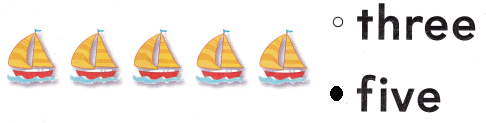# Texas Go Math Kindergarten Lesson 2.2 Answer Key Count and Write 5

Refer to our Texas Go Math Kindergarten Answer Key Pdf to score good marks in the exams. Test yourself by practicing the problems from Texas Go Math Kindergarten Lesson 2.2 Answer Key Count and Write 5.

## Texas Go Math Kindergarten Lesson 2.2 Answer Key Count and Write 5

Explore

DIRECTIONS: Count the cubes. Tell how many. Trace and write the numbers and the word. Count the apples. Tell how many. Write the numbers.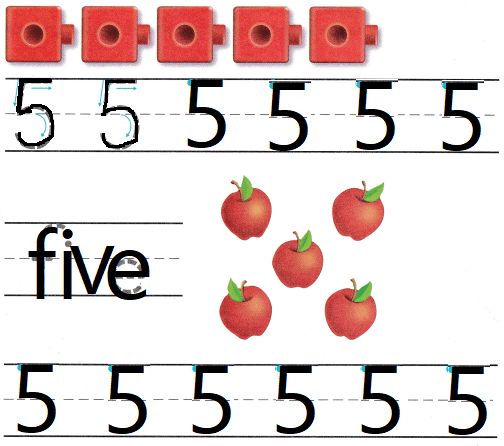Explanation:
Written the number 5 and the number name
there are 5 apples

Share and Show

DIRECTIONS: 1. Count and tell how many apples. Trace and write the numbers. 2. Circle the sets of five apples.

Question 1.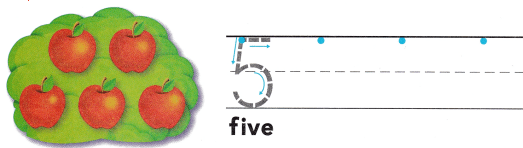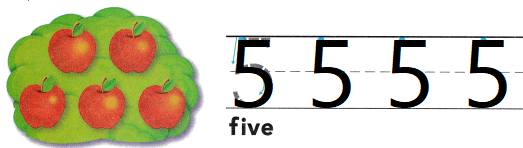Explanation:
Written the number 5 and
there are 5 apples

Question 2.Explanation:
Counted the apples and circled the group of 5 apples

DIRECTIONS: 3-6. Count and tell how many apples. Write the number.

Question 3.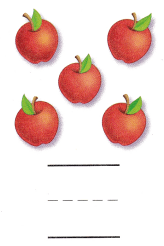Explanation:
There are 5 apples
and traced the number 5

Question 4.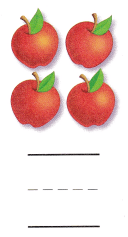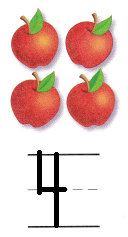Explanation:
There are 4 apples
and traced the number 4

Question 5.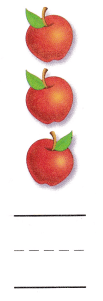Explanation:
There are 3 apples
and traced the number 3

Question 6.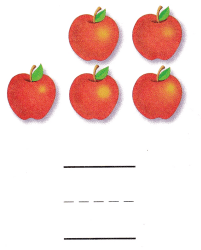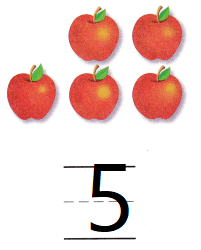Explanation:
There are 5 apples
and traced the number 5

HOME ACTIVITY • Ask your child to write the number 5 on a sheet of paper. Then have him or her find objects to show that number.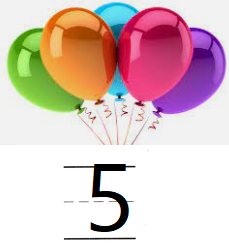Explanation:
Written the number 5 on a sheet of paper.
and counted 5 ballons

DIRECTIONS: 7. Frank found 5 rocks. Draw the rocks. Write the number. 8. Choose the correct answer. How many seashells?

Problem Solving

Question 7.Explanation:
There are 5 rocks
and written the number 5

Question 8.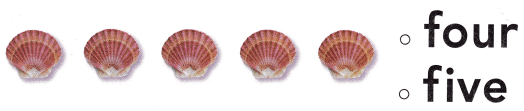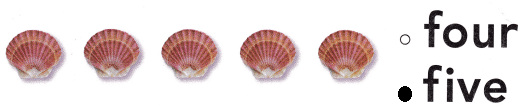Explanation:
There are 5 shells
and bubbled the number name 5

### Texas Go Math Kindergarten Lesson 2.2 Homework and Practice Answer Key

DIRECTIONS: 1-4. Count and tell how many trees. Write the number.

Question 1.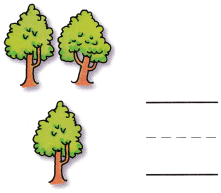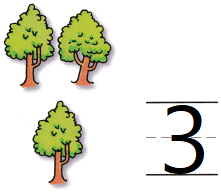Explanation:
There are 3 trees
counted and written the number

Question 2.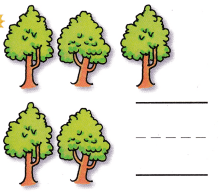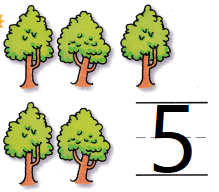Explanation:
There are 5 trees
counted and written the number

Question 3.Explanation:
There are 5 trees
counted and written the number

Question 4.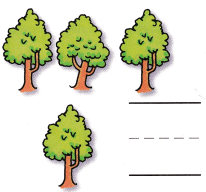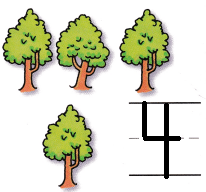Explanation:
There are 4 trees
counted and written the number

DIRECTIONS: Choose the correct answer. 5. How many pelicans? 6. How many birds? 7. How many sailboats?

Lesson Check

Question 5.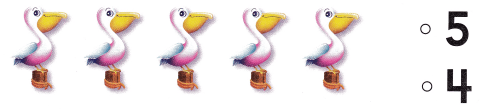Explanation:
There are 5 cranes
and bubbled the number 5

Question 6.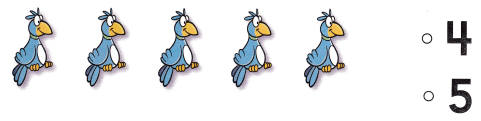Explanation:
There are 5 birds
and bubbled the number 5

Question 7.